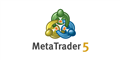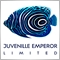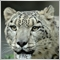# Need help with trigger sell positions70

Hi, any help is appreciated, I can't figure out why my EA only does buy positions.  I need it to do sell positions also, on multiple time frames simultaneously.

Here's the code:

```input int            MagicNumber  = 900;     //Magic Number
extern int           period_RSI   = 3,
exitsell     = 30;
input double         Lots         = 0.10;     //Fixed Lots
input double         StopLoss     = 250;      //Fixed Stop Loss (in Points)
input double         TakeProfit   = 250;      //Fixed Take Profit (in Points)
input int            TrailingStop = 0;       //Trailing Stop (in Points)
input int            k_period     = 5;        //Stochastic K Period
input int            d_period     = 2;        //Stochastic D Period
input int            slowing      = 3;        //Stochastic Slowing
input ENUM_MA_METHOD ma_method    = MODE_SMA; //Stochastic Moving Average Type
input int            price_field  = 0;        //Price field parameter. 0=Low/High or 1=Close/Close
input double         over_bought  = 80;       //Stochastic Over-Bought
input double         over_sold    = 20;       //Stochastic Over-Sold
//---
int    Slippage=5;
double sto_main_curr,RSI0,RSI1;

//+------------------------------------------------------------------+
//| Calculate open positions                                         |
//+------------------------------------------------------------------+
int CalculateCurrentOrders(string symbol)
{
//---
for(int i=0;i<OrdersTotal();i++)
{
if(OrderSymbol()==Symbol() && OrderMagicNumber()==MagicNumber)
{
if(OrderType()==OP_SELL) sells++;
}
}
//--- return orders volume
else       return(-sells);
}

//+------------------------------------------------------------------+
//| Check for open order conditions                                  |
//+------------------------------------------------------------------+
void CheckForOpen()
{
int    res;

sto_main_curr  = iStochastic (Symbol() ,PERIOD_CURRENT ,k_period ,d_period ,slowing ,ma_method ,price_field ,MODE_MAIN   ,0);

RSI0=iRSI(NULL,0,period_RSI,PRICE_CLOSE,0);
RSI1=iRSI(NULL,0,period_RSI,PRICE_CLOSE,1);

//--- sell conditions

if(RSI0 < sto_main_curr && RSI1 >= over_bought)
{
res=OrderSend(Symbol(),OP_SELL,Lots,Bid,Slippage,0,0,"",MagicNumber,0,FireBrick);
return;
}

if(RSI0 > sto_main_curr && RSI1 <= over_sold)
{
return;
}
//---
}
//+------------------------------------------------------------------+
//| Check for close order conditions                                 |
//+------------------------------------------------------------------+
void CheckForClose()
{

//--- get RSI
RSI0=iRSI(NULL,0,period_RSI,PRICE_CLOSE,0);
//---
for(int i=0;i<OrdersTotal();i++)
{
if(OrderMagicNumber()!=MagicNumber || OrderSymbol()!=Symbol()) continue;
//--- check order type
{
//here is the close buy condition
{
if(!OrderClose(OrderTicket(),OrderLots(),Bid,3,Black))
Print("OrderClose error ",GetLastError());
}
break;
}
if(OrderType()==OP_SELL)
{
// here is the close sell condition
if ((RSI0 < exitsell) )
{
Print("OrderClose error ",GetLastError());
}
break;
}
}
//---
}
//+------------------------------------------------------------------+
//| OnTick function                                                  |
//+------------------------------------------------------------------+
void OnTick()
{
//--- check for history and trading
return;
//--- calculate open orders by current symbol
if(CalculateCurrentOrders(Symbol())==0) CheckForOpen();
else                                    CheckForClose();
//---
}
//+------------------------------------------------------------------+```is an instruction given to a broker to buy or sell a financial instrument. There are two main types of orders: Market and Pending. In addition, there are special Take Profit and Stop Loss levels. is the commercial exchange (buying or selling) of a financial security. Buying is executed at the demand price (Ask), and Sell is performed at the...Moderator
10213882

Hello friend,

I am fairly sure that this part of your code is not helping.

```         if(OrderType()==OP_BUY)  buys++;
if(OrderType()==OP_SELL) sells++;```

The first if(statement) is executed, the second If(statement) is thrown out.70

GrumpyDuckMan:

Hello friend,

I am fairly sure that this part of your code is not helping.

The first if(statement) is executed, the second If(statement) is thrown out.

I believe it is somewhere in that part of the code, but not sure that particular part is the situation.70

Eleni Anna Branou:

okay. I don't see how to edit though.Moderator
20336

kamalagroup:
okay. I don't see how to edit though.

I have done it for you this time, next time please use the button that Eleni has shown you when posting code.Moderator
20336

GrumpyDuckMan:

Hello friend,

I am fairly sure that this part of your code is not helping.

```         if(OrderType()==OP_BUY)  buys++;
if(OrderType()==OP_SELL) sells++;```

The first if(statement) is executed, the second If(statement) is thrown out.

Not so,

both if conditions will be tested.70

Keith Watford:

Not so,

both if conditions will be tested.

That same code set up is used in many EA's I see.
My EA is not executing Sells, that part is calculating current orders.Moderator
20336

kamalagroup:
That same code set up is used in many EA's I see.
My EA is not executing Sells, that part is calculating current orders.

Yes, I know what it does.

I was responding to GrumpyDuckMan's post and his incorrect observation.882

Hello friends,

This code hasn't been tested.

```int CheckForOpen()
{
int    res; // make global

sto_main_curr  = iStochastic (Symbol() ,PERIOD_CURRENT ,k_period ,d_period ,slowing ,ma_method ,price_field ,MODE_MAIN   ,0);

RSI0=iRSI(NULL,0,period_RSI,PRICE_CLOSE,0);
RSI1=iRSI(NULL,0,period_RSI,PRICE_CLOSE,1);

//--- sell conditions

if(RSI0 < sto_main_curr && RSI1 >= over_bought)
{
res=OrderSend(Symbol(),OP_SELL,Lots,Bid,Slippage,0,0,"",MagicNumber,0,FireBrick);
return(res);
}

if(RSI0 > sto_main_curr && RSI1 <= over_sold)
{
return(res);
}
//---
}```

`stoploss=MarketInfo(NULL,MODE_STOPLEVEL)*_Point`

If you know the ticket number or magic number, why use select by pos?

`      if(OrderSelect(i,SELECT_BY_POS,MODE_TRADES)==false) break;`

I do something like this to close positions down.

```bool ClosePosition()
{
}```

this may be of some help also.

```bool Buy()
{

if(ticket<0)
{
return
(errorlevel=(GetLastError() ));
}
else errorlevel=(GetLastError() );
return(errorlevel);
}```

this way I can debug if OrderSend(); has successfully placed the order.882

Keith Watford:

Not so,

both if conditions will be tested.

```         if(OrderType()==OP_BUY)  buys++;
if(OrderType()==OP_SELL) sells++;```

Edited to include the code that is referred to.

Hello again,

The first if statement buy++ is always going to greater than the second if statement sell++.

True, or not?

```   if(buys>0) return(buys);
else       return(-sells);```
buys and -sells are only variables to a function.